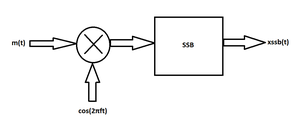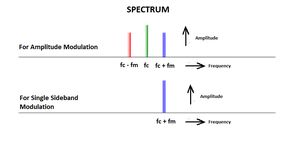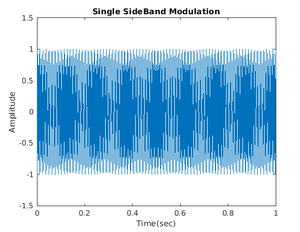Skip to content
Related Articles
Single Side Band (SSB) Modulation using MATLAB
• Last Updated : 20 Aug, 2020

Single sideband (SSB) modulation might is a sort of modulation used to send data, which includes an audio signal. Amplitude modulation produces an output signal in which the bandwidth of it is two times the most frequency of the original baseband signal. SSB modulation neglects this bandwidth increase, and also the power wasted on a carrier, at the price of more device complexity and tougher tuning at the receiver side.

Block Diagram :Spectrum :The Expression for Single Side Band with Upper Side Band :here,
m is the cosine wave with time duration of ‘t’,
fc is the carrier frequency,
t is the time duration and
mh s the Hilbert transform of baseband.

The MATLAB code is:

 % carrier frequencyfc = 200;  % baseband frequencyfm = 30;  % sampling frequencyfs= 4000;  % time durationt = (0 : 1 / fs :1 );t = linspace(0, 1, 1000);  % cosine wave with time duration of 't'm = cos(2 * pi * fm * t);   % Hilbert transform of basebandmh = imag(hilbert(m));   % Single Side Band with Upper Side Band sb = m .* cos(2 * pi * fc * t) - mh .* sin(2 * pi * fc * t);   % displaying the modulationfigure;plot(t, sb);title('Single SideBand Modulation');xlabel('Time(sec)');ylabel('Amplitude');

Output:My Personal Notes arrow_drop_up